# Engineering Mechanics | Solved Examples | Addition and Subtraction of Vectors

Example1: A person walks 300m to the east and 400m to the north to reach his friend’s house. What is the total displacement of the person, and what is the total distance traveled by him?

Recall that distance is a scalar quantity. Thus the total distance covered is 700m. Displacement, on the other hand, is a vector quantity so to find the net displacement; we add the two vectors to get a displacement of 500m at an angle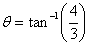from east to north (Figure 7).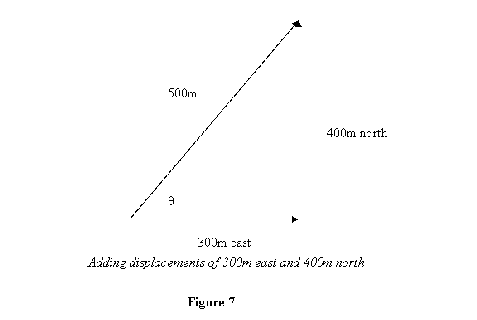Example 2 : Two persons are pushing a box so that the net force on the box is 12N to the east If one of the person is applying a force 5N to the north, what is the force applied by the other person.

Let the force by person applying 5N be denoted by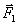and that by the other person by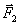. We then have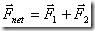so that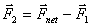Solution foris given graphically in figure 8. The force comes out to be 13N at an angle of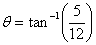from east to south.

Figure 8